# GED Math : Statistics

## Example Questions

### Example Question #51 : Statistics

There are 12 marbles in a box. There are 5 blue, 2 green, 3 yellow, and 2 red. Two marbles are selected, WITH replacement. What is the probability of selecting one red and one yellow?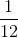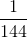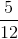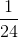Explanation:

You can select red and yellow in one of two ways. That is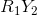OR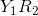.

It is key, in this problem, to recall that the selection is WITH replacement.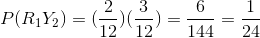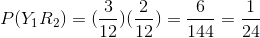If we add the two probabilities, we get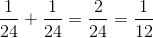.

### Example Question #51 : Statistics

What is the probability of selecting a king from a deck of cards, putting it aside, and then selecting an ace?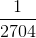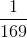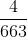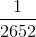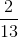Explanation:

This question involves probability WITHOUT replacement.

In a regular deck of cards, there are 52 cards, with 4 kings. The probability of selecting a king is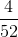. Once that card is out of the deck, there are 51 cards left. There are 4 aces out of 51 cards. The probability of selecting an ace out of 51 cards is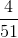.

The probability of selecting a kind AND an ace is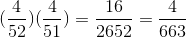### Example Question #53 : Probability

Two regular 6-sided dice are thrown. What is the probability of rolling a sum of 8?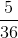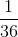Explanation:

There are 36 equal outcomes when rolling two dice. The outcomes which fit the criteria of a "sum of 8"  are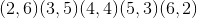. The are 5 such outcomes.

The probability of rolling a sum of 8 is.

### Example Question #53 : Calculations

How many different ice cream cones can be made using one of each of the following:

Flavor: Vanilla, Chocolate, Strawberry, Cookie Dough

Topping: Rainbow Sprinkles, Chocolate Sprinkles, Almonds, Chocolate Chips, Peanut Butter Cups

Cone Type: Waffle, SugarExplanation:

This question calls for the use of the counting principle. The counting principle has one multiply the number of options in each category. In this case, there are 4 options for flavors, 5 options for toppings, and 2 options for cone type.

So,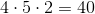There are 40 different ice cream cones to be made using one in each category.

### Example Question #51 : Calculations

On a movie and show night, a family plans on watching two shows and one movie. Consider the following:

1) The family has five shows that they really want to see, but decide that two will work.

2) After the shows, they will choose from one of seven DVD movies that they all agree on.

How many ways can the family watch two out of the five shows AND one of the seven movies on that night?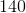Explanation:

This employs the counting principle, much in the way one might be used to seeing.

The family has a combination of 2 out of the 5 shows AND 1 of the 7 movies.

We call the shows A, B, C, D, and E AND the movies 1,2,3,4,5,6, and 7.

1) Choosing two shows, we have {AB, AC, AD, AE, BC, BD, BE, CD, CE, DE}. The are 10 of these.

Choosing one movie, we have {1,2,3,4,5,6,7} There are 7 of these.

There are 10 "sets" of shows and 7 "sets" of movies.

Therefore, by the counting principle, the family has 10 x 7 options to choose from. There is a total of 70 options.

2) Another way is taking multiplying the combinations of shows and movies.

That is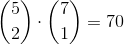### Example Question #51 : Statistics

A weighted coin is flippedtimes.  It is weighted such that heads comes upout oftimes on average.  What is the probability that the person in question will flip all tails?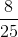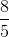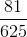Explanation:

If the weighting isfor heads, this means that it isfor tails.  Therefore, the probability of flippingtails will be:### Example Question #1 : Mean

Find the mean of the following numbers: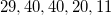Explanation: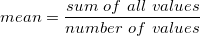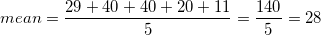### Example Question #1 : Mean

Find the mean of the set of numbers below: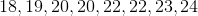Explanation: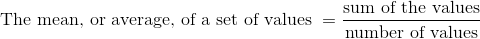In this list,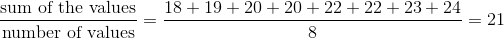### Example Question #3 : Mean

Given the data set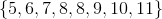, which of the following quantities are equal to each other/one another?

I: The mean

II: The median

III: The mode

II and III only

I, II, and III

I and II only

I and III only

I, II, and III

Explanation:

The mean of a data set is the sum of its elements divided by the number of elements, which here is 8: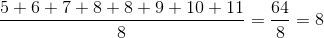The median of a data set with an even number of elements is the mean of the middle two values when the set is arranged in ascending order; both of the middle elements are 8, so the median is 8.

The mode of a data set is the most frequently occurring element. Here, only 8 appears twice, so it is the mode.

All three are equal.

### Example Question #1 : Mean

Veronica scored a 75 and a 71 on her first two exams. To raise her mean score to an 80, what grade does she need to earn on her third test?

73

80

90

94

85

94

Explanation:

The mean score is the sum of all scores divided by the number of scores.

First, find the sum of all three test scores, then divide by the number of tests, 3.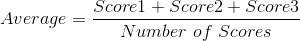If Veronica wants her average to be 80, the equation becomes: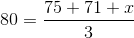We need to solve for.

First, multiply both sides of the equation by 3: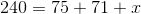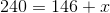Subtract 146 from both sides: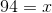Veronica must score a 94 on her third test in order to bring her mean score to 80.

### All GED Math Resources